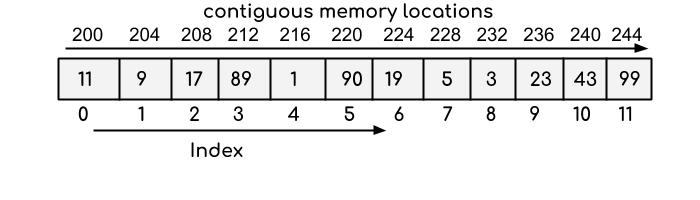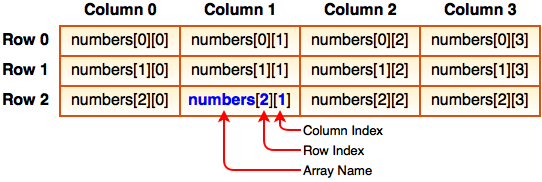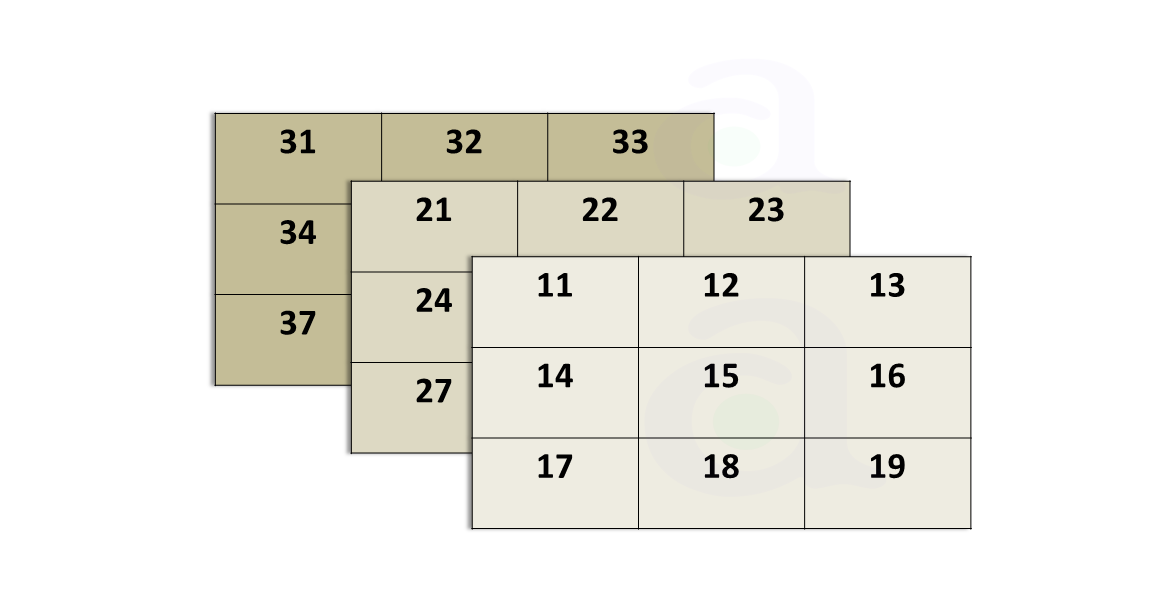## Introduction to Arrays

Having understood the various data types, taking inputs from console and displaying it on console let's move to arrays.

What is an array?

An array is a collection of similar kind of elements which are located in the memory sequentially. An array index starts from 0 and ends depending on length of array.

Below is an example of an integer array of size 12 where array index starts at 0 and ends at 11 ( Array size -1 ). Here, it is an array of type “integer” which means all integer elements are inserted into the array. As integer takes 4 bytes of memory, each memory allocation differs by 4 bytes.Similarly, if we have taken a character array ,each memory is allocated with 1 byte and have elements as the characters and it is similar for all remaining other data types and each memory size depends upon the data type we are using.
We have two types of Arrays:

1. Static Arrays.
2. Dynamic Arrays

We first look into the static arrays

Static array is an array in which memory is loaded at compile time i.e, we provide the size of an array or insert the elements in an array before compile time. Once we define the size of an array, it is not possible to increase or decrease the size of an array.
You may ask that what if we are accessing an element beyond size of the array?

Ans: You may get array index out of bounds error or any garbage value that is stored in that particular memory location

Single Dimensional Array or 1-D Array:

It is an array consisting of ‘n’ number of elements stored in linear fashion.

Syntax:

<data type> <variable_name> [ size ];

Initializing 1-D Array:

If we mention people={0}, it doesn’t mean that all array elements are initialized to zero, only the starting element is initialized with zero i.e, ( people=0 ).

int a;
int a[]={ 34, 56 , 12 , 26, 78 };

The first example explains that it is an integer array of size 100 with no initalization.
The second example explains that it is an integer array where the array elements are 34,56,12,26 and 78.

You may ask that the array size is not mentioned so it is not static array.
Here, the size of the array is precalculated before compile time by knowing the number of elements that are intialized in the array.

size_of_array = sizeof(people) / sizeof(people);

"sizeof" is a keyword which calculates the size of the data type we need.

Try it yourself for remaining other data types !

1. Double Dimensional Arrays or 2-D Arrays :

It is an array where we can allocate the elements in the form of rows and columns.

Syntax in C++:
<data_type> <variable_name>[size][size];Initializing 2-D Array:

int table;
The first example is an array containing 2 rows and 3 columns with no initializaion.

int table={{2,3,4},{7,8,9}};
The second example is an array containing 2 rows and 3 columns where 1 st row contains elements {2,3,4} and 2nd row contains elements {7,8,9}.

Invalid Declarations of 2-D array:

int table={2,3,4,7,8,9};
int tab[]={2,3,4,7,8,9};

Try it Yourself for remaining other data types !

1. Multi Dimensional Arrays:

It is an array where we can allocate the elements in the form of tables,rows and columns.

Syntax:

<data_type> <variable_name>[size][size]…[size];Initializing Multi Dimensional Array:

float display;
The first example is a multi dimensional arrayof type float with no initialization.

float display={{{1.5,2.3},{3.5,7.6}},{{3.2,4.6},{5.8,9.3}}};
The second example is a multi dimensional array with initialization as follows :

display=1.5;

display=2.3;

display=3.2;

display=4.6;

Invalid Declarations of N-D array:

display={{{1,2}},{{1,2}}};
This above example throws array index out of bounds error because it consists of only 1 table with 2 rows and 2 columns but the right side elements are written according to 2 tables of 1 row and 2 columns.

• Efficient execution time

• We can access any element within the array size in O(1) time complexity

Think about how we can access element at index 2 within array of size 4 ?

• We can modify any content within array size in just O(1) time complexity. As, we can access any index within the array.

• As the size is fixed ,we cannot insert elements beyond the array size.

• Time complexity of inserting an element is O(size_of_array).

• Generally, Time complexity for searching an element in array is O(size_of_array).

We can optimise it,by using other searching algorithms.

• Removing an element in the array is also O(size_of_array).

For getting more grip over arrays ,try solving these problems

Happy Coding 😊

By Programmers Army

Contributed by: Ravi Teja Chidurala